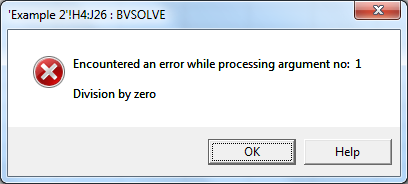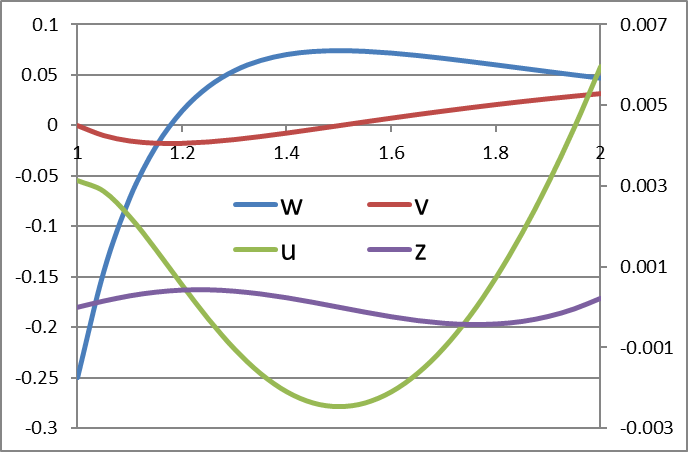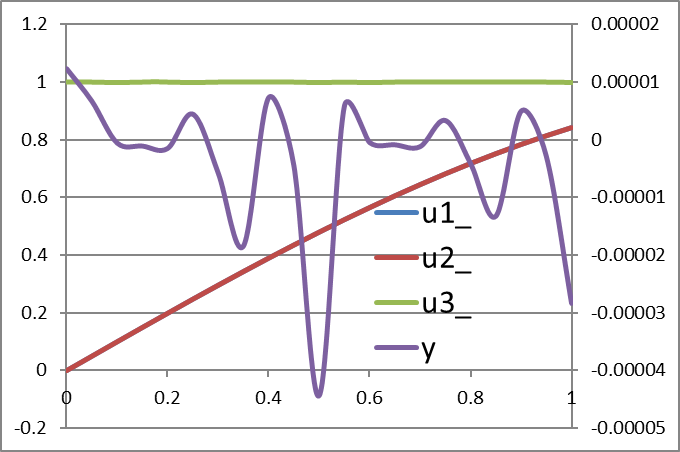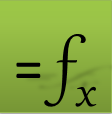=BVSOLVE(eqns, vars, bcs, domain, [options])

Use BVSOLVE to solve a boundary-value ODE or DAE system in the form: (the system can have as many equations as needed)

Example: $\frac{d{y}_{1}}{dx}={f}_{1}\left(x,{y}_{1},{y}_{2},{y}_{3}\right)$ $\frac{d{y}_{2}}{dx}={f}_{2}\left(x,{y}_{1},{y}_{2},{y}_{3}\right)$ With optional one or more algebraic equations: $0={g}_{1}\left(x,{y}_{1},{y}_{2},{y}_{3}\right)$

Boundary Conditions

To complete description of the problem, boundary conditions implicating the differential variables are required. General nonlinear boundary conditions can be specified at any location within the the system spatial domain [xs, xe].

Required Inputs

eqns a reference to the system formulas (f1, f2,..,g1,..).

vars a reference to the system variables in the following order (x, y1, y2,.. ).

Optional. You can define starting guess for (y1, y2,.. ). The guess can be a constant or a function of x.

bcs A reference to a 2-column array defining the boundary conditions. The first column specifies boundary conditions points, and the 2nd column specifies corresponding boundary condition formulas. The boundary conditions points must be ordered from left to right.

Boundary condition points may be repeated if multiple boundary conditions are specified at the same location.

A boundary condition formula is arranged with respect to zero on one side. For Example, suppose that the boundary condition (BC) at x0 is y1= y2+1, then the BC formula may be defined as =Y1-Y2-1.

domain a vector defining the start and end values for the spatial domain. Additional optional data controls the output spatial values in the computed solution as described below.

FormatRemarks
{xs, xe}The domain [xs, xe] is divided uniformly according to the number of allocated rowsin the output array.
{{xs, xe, ndiv}The domain [xs, xe] is divided uniformly into ndiv subintervals.The allocated output array must have sufficient rows to hold the result.
{xs, x1, x2, .., xe}Solution results are reported for the supplied values only. The allocated output array must have sufficient rows to hold the result.

Optional Inputs

m the number of algebraic equations for a DAE system.

For a DAE system, algebraic equations and variables should be listed last in eqns and vars.

rtol relative error tolerance. you may specify a fixed value for all variables, or supply vector of tolerances for each variable in vars. Default is 1.0e-4.

ctrl a set of key/value pairs for algorithmic control as detailed below.

Description of key/value pairs for algorithmic control

 Key MAXMESH Default Value 1000 (Integer) Remarks Sets an upper bound on the mesh size for the spatial domain.

 Key INITMESH Default Value 7 (Integer) Remarks Sets the initial mesh size which is refined adaptively during iterations.

 Key KORDER Admissible Values (Integer) 2 ≤ KORDER ≤ 7 Default Value 4 Remarks No. of collocation points per mesh subinterval. A higher value will increase computational cost.It may help with a highly oscillating system.

 Key LINEAR Admissible Values (Boolean) True or False Default Value False Remarks Set to TRUE if the problem is linear with linear boundary conditions

 Key ERROREST Admissible Values (Boolean) True or False Default Value False Remarks Set to true to report global error estimates for the differential variables in the solution output array.The last row of the allocated output array will be reserved for the error estimates.
 Key JACSTEP Admissible Values (Real) 0 < JACSTEP < 0.1 Default Value The default step is computed dynamically based on machine accuracy, preset limits and function metric. For an order(1) function it is approximately 1.0e-8. Remarks The default value generally produces accurate approximations; however relaxing the default value may aid convergence on some problems with unknown Jacobian such as parameterized problems.

 Key JACSCHEME Admissible Values (Integer) 1 for First order Euler forward scheme2 for Second order Euler forward scheme Default Value 1 Remarks First order scheme is generally sufficient.

sysjac a reference to the system analytical Jacobian matrix for the functions (f1, f2,..,g1,..) with respect to the variables (y1, y2,.. ).

For a system with two functions, the Jacobian matrix has the following order: $\begin{array}{cc}\frac{\partial {f}_{1}}{\partial {y}_{1}}& \frac{\partial {f}_{1}}{\partial {y}_{2}}\\ \frac{\partial {f}_{2}}{\partial {y}_{1}}& \frac{\partial {f}_{2}}{\partial {y}_{1}}\end{array}$

bcjac a reference to the boundary conditions analytical Jacobian matrix with respect to the differential variables only (y1, y2,.. ).

BVSOLVE is evaluated as an array formula in an allocated range of the spreadsheet. It computes and displays a formatted solution as illustrated in Figure 1 for a system with two state variables and a spatial domain [0 ,1]. The first column displays the spatial points, and the subsequent columns display the corresponding values of the state variables.

 A B C 1 x y1 y2 2 0 y1(0) y2(0) 3 0.1 ↓ ↓ 4 0.2 .. ↓ 12 1 y1(1) y1(1) 13 Rel. Error error y1 error y2

When allocating a range for BVSOLVE, the following rules apply:

• The number of allocated columns should match the number of variables.

• The number of allocated rows is arbitrary. It determines the increment for the domain subdivisions (only for display purpose).
You can customize the output spatial points using any of the following formats in argument number 5.

FormatRemarks
{xs, xe}The domain [xs, xe] is divided uniformly according to the number of allocated rowsin the output array.
{{xs, xe, ndiv}The domain [xs, xe] is divided uniformly into ndiv subintervals.The allocated output array must have sufficient rows to hold the result.
{xs, x1, x2, .., xe}Solution results are reported for the supplied values only. The allocated output array must have sufficient rows to hold the result.
• If the control key ERROREST value is set to True (see examples below), the last row in the allocated output array will display the estimated maximum global error for each variable.

Consider the following 2nd-order nonlinear differential equation in the spatial domain [0,1]

with end points boundary conditions:

Solution

Using standard substitution technique we convert the 2nd-order equation to two 1st-order equations

$\frac{du}{dx}=z$

Working with the named variables x, y, z, and eps for cells B2:B5, we define the system equations and boundary conditions as shown in Table 1 below. The colored ranges represent the required input arguments to BVSOLVE. They are: the RHS-side formulas in B7:B8, the selected system variables B2:B4 (named x, y, z,), and the boundary conditions matrix C2:D3.

 Variables BCs Equations A B C D 1 2 x 0 =u 3 u 1 =u 4 z 5 eps 0.01 6 7 du/dx =z 8 dz/dx =(-EXP(u)*z+0.5*PI()*SIN(0.5*PI()*x)*EXP(2*u))/eps

Next, we evaluate the array formula =BVSOLVE(B7:B8, (x,y,z), C2:D3, {0,1}, , , {"ERROREST", True}) in an allocated array G4:I25 and obtain the solution shown in Table 2 below.

• We have used Excel union operator, (), to combine the system variables x,y,z into one reference for argument 2. Alternatively, we could pass the raw address B2:B4 instead.
• We have used Excel constant array syntax, {}, to pass the spatial domain [0,1] in argument 4.
• Note that we skip over optional parameters 5 and 6 and pass the key ERROREST in parameter 7 to report global error estimates in the last row of the output array.
The obtained result is plotted in Figure 1.

 G H I 4 x u z 5 0 0 -9.22395 6 0.05 -0.3043492 -3.93625 7 0.1 -0.44424441 -1.92551 8 0.15 -0.51353621 -0.95066 9 0.2 -0.54647914 -0.41601 10 0.25 -0.55866051 -0.09636 11 0.3 -0.55796756 0.110589 12 0.35 -0.5486075 0.256436 13 0.4 -0.53287428 0.368873 14 0.45 -0.51201677 0.463304 15 0.5 -0.48669581 0.548502 16 0.55 -0.45723449 0.629561 17 0.6 -0.42375759 0.709506 18 0.65 -0.38627075 0.790212 19 0.7 -0.34470297 0.872914 20 0.75 -0.29893093 0.958517 21 0.8 -0.24879048 1.047768 22 0.85 -0.19408162 1.14137 23 0.9 -0.13456902 1.240042 24 0.95 -0.06997994 1.344568 25 1 0 1.455833 26 Rel. Errors 1.87103E-06 8.35E-05

Optional Parameters

For demonstration purpose, we show how to define and pass the optional system and boundary conditions jacobians, well as custom tolerances. (In practice, passing in analytical Jacobians is unnecessary as the internal numerical approximations by the solver is usually accurate and sufficient.) We define the system jacobian in D8:E9, and the boundary conditions jacobian in D11:E12 as shown in Table 3 below.
The jacobian definition must respect the order of state variables, u, z as specified in argument 2 for the solver.

 System Jacobian BCs Jacobian D E 7 8 0 1 9 =-(EXP(u)*z-PI()*SIN(PI()*x/2)*EXP(2*u))/eps =-EXP(u)/eps 10 11 1 0 12 1 0

We evaluate the updated formula
=BVSOLVE(B7:B8, (x,y,z), C2:D3, {0,1}, 0, {0.0001,0.00001}, {"ERROREST",True}, D8:E9, D11:E12)
to obtain the new solution. Given that we have only 2 state variables, it is convenient to use an array constant to pass the tolerances vector in argument 6. The new results are essentially the same as the default results shown above in Table 2.

Consider the following stiff nonlinear 2nd-order differential equation in the domain [0,1]
$A\left(x\right)=1+{x}^{2}$ with end points boundary conditions

Using standard substitution technique we convert the 2nd order equation to two 1st order equations

$\frac{dy}{dx}=z$

Solution

We solve the above stiff system for $\epsilon =0.01$.

The solution steps are similar to Example 1. We work with the named variables, x, y, z, A, dA, eps corresponding to cells B2:B7 to define the system formulas and boundary condition matrix as shown in Table 1 below.

 Variables BCs Equations A B C D 1 2 x 0 =y-0.9129 3 y 1 =y-0.375 4 z 5 A =1+x^2 6 dA =2*x 7 eps 0.01 8 9 dy/dx =z 10 dz/dx =((2.4/2-eps*dA)*y*z-z/y-dA/A*(1-0.4/2*y^2))/(eps*A*y)

Next, we evaluate the array formula =BVSOLVE(B9:B10, (x,y,z), C2:D3, {0,1}, , , {"ERROREST", True}) in an allocated array H4:J26 to obtain the solution. However, the solver displays a fatal error message instead:Explanation

An error message can often be quite helpful. The caption of the window indicates the cell and solver that has caused the error, and the message often conveys details about the specific argument responsible for the error.
In this case, the error is in argument 1 which holds the system equations. Clearly, the solver encountered division by zero while evaluating one of the system equations. A quick inspection shows that formula B10 involves division by the variable y which has a default starting guess of zero. To fix this error, we simply define a non-zero starting guess of 1 for y in cell B3. The solver now converges successfully and produces the following result (Table 2) which is plotted in Figure 1.

 H I J 4 x y z 5 0 0.9129 0.835223 6 0.05 0.954646 0.834019 7 0.1 0.996237 0.828936 8 0.15 1.037468 0.819592 9 0.2 1.078129 0.806201 10 0.25 1.118026 0.789141 11 0.3 1.15699 0.7689 12 0.35 1.194872 0.745969 13 0.4 1.231542 0.72026 14 0.45 1.26676 0.684865 15 0.5 1.298649 0.549927 16 0.55 1.305552 -0.73972 17 0.6 1.083584 -10.5522 18 0.65 0.603002 -2.53229 19 0.7 0.547885 -0.76692 20 0.75 0.511759 -0.68353 21 0.8 0.479252 -0.6188 22 0.85 0.449708 -0.56442 23 0.9 0.422687 -0.51745 24 0.95 0.397869 -0.47612 25 1 0.375 -0.43931 26 Rel. Errors 8.33E-07 0.000237

This equation models a uniformly loaded beam of variable stiffness simply supported at both ends.

Using standard substitution technique we convert the 4th order equation to four 1st order equations.

Let

$dwdx=1-6x2w-6xvx3$ $dvdx=w$ $dudx=v$ $dzdx=u$

The equivalent boundary conditions are

Solution

The solution procedure is similar to Example 1 above. Working with the named variables x,u,v,w and z for cells B2:B6, we define the system equations and boundary conditions as shown in Table 1 below.

Table 1
 Variables BCs Equations A B C D 1 2 x 1 =z 3 u 1 =v 4 v 2 =z 5 w 2 =v 6 z 7 8 dw/dx =(1-6*x^2*w-6*x*v)/x^3 9 dv/dx =w 10 du/dx =v 11 dz/dx =u

To obtain the solution, We evaluate the array formula =BVSOLVE(B8:B11, B2:B6, C2:D5, {1,2}, , , {"ERROREST", True}) in allocated array H4:L26. The results are shown in Table 2 and plotted in Figure 1 below.

 H I J K L 4 x w v u z 5 1 -0.5 1.55242E-30 0.017132 0 6 1.05 -0.33011 -0.02051609 0.016584 0.000847162 7 1.1 -0.20832 -0.03380919 0.0152 0.001644528 8 1.15 -0.12078 -0.04191665 0.013289 0.002358447 9 1.2 -0.05787 -0.04629629 0.011071 0.002968346 10 1.25 -0.0128 -0.048 0.008704 0.003463059 11 1.3 0.019257 -0.04779245 0.006302 0.003838168 12 1.35 0.041773 -0.04623279 0.003947 0.004094076 13 1.4 0.057268 -0.04373178 0.001695 0.004234597 14 1.45 0.067583 -0.04059207 -0.00042 0.004265921 15 1.5 0.074074 -0.03703704 -0.00236 0.004195851 16 1.55 0.077746 -0.03323151 -0.00412 0.00403324 17 1.6 0.079346 -0.02929688 -0.00568 0.003787576 18 1.65 0.079432 -0.02532209 -0.00704 0.003468679 19 1.7 0.078423 -0.02137187 -0.00821 0.003086471 20 1.75 0.076635 -0.01749271 -0.00918 0.002650819 21 1.8 0.074303 -0.01371742 -0.00996 0.002171412 22 1.85 0.071605 -0.01006851 -0.01056 0.001657686 23 1.9 0.068677 -0.00656072 -0.01097 0.00111876 24 1.95 0.065617 -0.00320302 -0.01121 0.0005634 25 2 0.0625 1.73472E-18 -0.01129 -1.0842E-19 26 Rel. Errors 3.17E-07 4.16988E-08 5.59E-09 8.15553E-10

Interior boundary conditions

We can easily study the effect of the beam support locations. We solve the same problem but with the beam supported at left end and center (x=1 and x=1.5) with the right end free. The only required change to the problem setup is to change the values of the boundary points in cells C4 and C5 in Tabe 1 from 2 to 1.5. We re-run the solver and obtain the new solution plotted below.Consider the following differential algebraic (DAE) system of equations $du1dx=ε+u2-sin⁡(x)y+cos(x)$ $du2dx=cos(x)$ $du3dx=y$ $0=u1-sin⁡(x)*(y-ex)$

With boundary conditions

For small values of $ε$, this problem has the following solution

(See Ascher and Spiteri. Collocation software for boundary value differential-algebraic equations. SIAM Journal on Scientific Computing. Volume 15 938-952. 1995)

Solution

Working with the named variables x, u1_, u2_, u3_, y and eps for cells B2:B7, we define the system equations and boundary conditions as shown in Table 1 below.

 Variables BCs Equations A B C D 1 2 x 0 =u1_-SIN(0) 3 u1_ 0 =u3_-1 4 u2_ 1 =u2_-SIN(1) 5 u3_ 6 y 7 eps 0.001 8 9 du1/dx =(eps+u2_-SIN(x))*y+COS(x) 10 du2/dx =COS(x) 11 du3/dx =y 12 0 =(u1_-SIN(x))*(y-EXP(x))

To obtain the solution, We evaluate the array formula =BVSOLVE(B9:B12, B2:B6, C2:D4, {0,1}, 1) in allocated array H4:L26 and obtain the results shown in Table 2 below.

• The first argument B9:B12 is a reference to the system RHS formulas. Note that the algebraic equation B12 is ordered last as required for a DAE system.

• The 2nd argument B2:B6 is a reference to the system variables. Alternatively, we could pass the equivalent defined names by combining them with Excel union operator as follows (x, u1_, u2_, u3_, y). Note that the algebraic variable B6 (y) is listed last as required for a DAE system.

• We used Excel array constant syntax to pass the spatial domain [0, 1] in argument 4.

• We used optional argument 5 to tell BVSOLVE that the last equation in B9:B12 is an algebraic equation.

 H I J K L 4 x u1_ u2_ u3_ y 5 0 0 0 1 1.238E-05 6 0.05 0.049979 0.049979 1 6.752E-06 7 0.1 0.099833 0.099833 1 -4.951E-07 8 0.15 0.149438 0.149438 1 -1.035E-06 9 0.2 0.198669 0.198669 1 -1.459E-06 10 0.25 0.247404 0.247404 1 4.503E-06 11 0.3 0.29552 0.29552 1 -5.653E-06 12 0.35 0.342898 0.342898 1 -1.840E-05 13 0.4 0.389418 0.389418 1 7.336E-06 14 0.45 0.434966 0.434966 1 -4.392E-06 15 0.5 0.479426 0.479426 1 -4.436E-05 16 0.55 0.522687 0.522687 1 5.825E-06 17 0.6 0.564642 0.564642 1 -4.189E-07 18 0.65 0.605186 0.605186 1 -8.164E-07 19 0.7 0.644218 0.644218 1 -1.141E-06 20 0.75 0.681639 0.681639 1 3.401E-06 21 0.8 0.717356 0.717356 1 -4.072E-06 22 0.85 0.75128 0.75128 1 -1.321E-05 23 0.9 0.783327 0.783327 1 4.991E-06 24 0.95 0.813416 0.813416 1 -2.817E-06 25 1 0.841471 0.841471 1 -2.832E-05BVSOLVE is based on the COLDAE collocation method with adaptive mesh refinement.

Question or Comment? Email us:
support @ excel-works.comExceLab: Transforming Excel into a Calculus Power House
ExceLab functions and methods are protected by USA Patents 9286286, 9892108, 10114812 and pending.
© 2015-2019, ExcelWorks LLC
Boston, USA